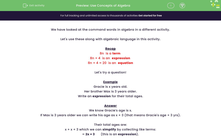# Use Concepts of Algebra

In this worksheet, students will use algebraic concepts using mathematical language.Key stage:  KS 3

Curriculum topic:   Algebra

Curriculum subtopic:   Use and Interpret Algebraic Notation

Difficulty level:#### Worksheet Overview

We have looked at the command words in algebra in a different activity.

Let's use these along with algebraic language in this activity.

Recap

8n  is a term
8n + 4  is an  expression
8n + 4 = 20  is an  equation

Let's try a question!

Example

Gracie is x years old.

Her brother Max is 3 years older.

Write an expression for their total ages.

We know Gracie's age is x.

If Max is 3 years older we can write his age as x + 3 (that means Gracie's age + 3 yrs).

Their total ages are:

x + x + 3 which we can simplify by collecting like terms:

= 2x + 3       (this is an expression).Part 2

If we knew that the combined ages of Gracie and Max is 25 years, we could make an equation with the expression we have:

2x + 3 = 25

We could then solve this to find Gracie's age (x).

2x = 22

x = 11

Therefore, Gracie is 11 years old and Max is 3 years older, so he is 11 + 3 = 14 years old.

Let's try some of these!

### What is EdPlace?

We're your National Curriculum aligned online education content provider helping each child succeed in English, maths and science from year 1 to GCSE. With an EdPlace account you’ll be able to track and measure progress, helping each child achieve their best. We build confidence and attainment by personalising each child’s learning at a level that suits them.

Get started